Chen, X., Xie, M., Wang, H., Xue, Y., and Li, Z. (2014). "Preparation of silica by alkaline-heating and precipitation method from corn straw ash," BioRes. 9(4), 6719-6734.

#### Abstract

The preparation of silica by an alkaline-heating and precipitation method from corn straw ash (CSA) obtained from a biomass power plant was the focus of this study. Sodium hydroxide was used as the silica extraction reagent, and H2SO4 was the precipitator. The concentration of sodium hydroxide solution was confirmed to have a significant influence on the production of sodium silicate from CSA. The optimum technological parameters for the preparation of sodium silicate and the suitable parameters for the preparation of silica were obtained by experiments and analysis. Fourier transform infrared spectroscopy (FT-IR) was used for confirming that the as-obtained silica has characteristic IR absorption peaks of typical hydrated silicon dioxide. The microstructures of the as-obtained silica products were characterized by scanning electron microscopy (SEM) and transmission electron microscopy (TEM). Comparison using HG/T3061 (2009) standards, and the characteristics of silica products were used to estimate whether the as-obtained silica products reached market requirements. The results show that the silica products are superior to the standard requirements and have a large market potential.

#### Full Article

Preparation of Silica by Alkaline-Heating and Precipitation Method from Corn Straw Ash

Xudong Chen,* Ming Xie, Huaibin Wang, Yingxi Xue, and Zhengqi Li

The preparation of silica by an alkaline-heating and precipitation method from corn straw ash (CSA) obtained from a biomass power plant was the focus of this study. Sodium hydroxide was used as the silica extraction reagent, and H2SO4 was the precipitator. The concentration of sodium hydroxide solution was confirmed to have a significant influence on the production of sodium silicate from CSA. The optimum technological parameters for the preparation of sodium silicate and the suitable parameters for the preparation of silica were obtained by experiments and analysis. Fourier transform infrared spectroscopy (FT-IR) was used for confirming that the as-obtained silica has characteristic IR absorption peaks of typical hydrated silicon dioxide. The microstructures of the as-obtained silica products were characterized by scanning electron microscopy (SEM) and transmission electron microscopy (TEM). Comparison using HG/T3061 (2009) standards, and the characteristics of silica products were used to estimate whether the as-obtained silica products reached market requirements. The results show that the silica products are superior to the standard requirements and have a large market potential.

Keywords: Corn straw ash; Alkaline-heating; Precipitation; Sodium silicate; Silica

Contact information: College of Energy Science and Engineering, Harbin Institute of Technology, No. 92, West Dazhi Street, Harbin 150001, China; *Corresponding author: 19851025cxd@163.com

INTRODUCTION

Silica is a basic raw material that is widely used in the electronics, ceramics, and polymer material industries (An et al. 2010; Li and Wu 2012). Usually, because of its high value and favorable performance, silica prepared by the gas phase method is used in silicone rubber and some special products (Yan et al. 2010); the most important application of silica prepared by the precipitation method is as reinforcing filler (Li 2010). As a reinforcing agent, silica can improve the hardness, tensile strength, tear strength, and wear resistance in rubber and plastic products. Additional applications of silica include liquid carrier, flow agent, anti-caking agent, friction agent, dispersing agent, anti-settling agent, and extinction agent (Kohls and Beaucage 2002; Li et al. 2011).

Corn straw is an abundant agricultural byproduct available worldwide. Studies on corn straw have been conducted, to include improving the nutritional value of corn straw as animal feed (Gao et al. 1997), kinetic studies of corn straw pyrolysis (Li et al. 2008), combustion characteristics of different parts of corn straw (Zhao et al. 2008), production of biogas from corn straw (Zhong et al. 2011), influence of pesticide contamination to the land from open burning of corn straw (Zhang et al. 2011), and pretreatment optimization for fermentation of corn straw hydrolyzates (Zhang et al. 2012).

Corn straw ash (CSA) is the waste product from burning corn straw as fuel. At present, CSA is not utilized adequately. In some areas, large amounts of CSA are treated as waste and disposed of in landfill sites. Questions have been raised regarding the environmental impact, resulting in the necessity for studies on the legitimate utilization of CSA. Several studies on the technology for the production of compound fertilizer from CSA have been conducted, including those by Huang et al. (2006) and Li et al. (2007). Binici and Aksogan (2011) studied the use of ground blast furnace slag, chrome slag, andCSA mixtures as a coating against corrosion, and Yue and Jiang (2009) studied slag cement admixtures with CSA. Other investigators (Xu et al. 2008; Ju 2010) studied methods for extracting potassium from CSA.

Liu et al. (2011; 2012) studied the preparation of silica and activated carbon from rice husk ash. Previous studies (Huang et al. 2006; Li et al. 2007; Xu et al. 2008; Yue and Jiang 2009; Ju 2010) showed that CSA is rich in silica, at over 60%, with few mineral impurities. Because CSA has few mineral impurities, the purification of silica from CSA is easier than the purification of silica from minerals. Additionally, the exploitation of minerals can be expensive, and CSA is a waste product and thus inexpensive. Hence, CSA is an economically viable raw material for the production of silica.

This study investigated the preparation of silica by an alkaline-heating and precipitation method from CSA. The effects of various factors on the dissolution rate of silica and the sodium silicate modulus, the technological parameter optimization for the production of sodium silicate from CSA, the effects of various factors on the purity of silica, and some characteristics of silica products were determined.

EXPERIMENTAL

Materials

Corn straw ash (CSA) was obtained from a biomass power plant in Heilongjiang Province, China.

Reagents

All chemical reagents, including sodium hydroxide, hydrochloric acid, anhydrous alcohol, bromocresol green, methyl red, sodium fluoride, sulfate (sulfuric) acid, ethylenediaminetetraacetic acid, disodium salt (EDTA-Na2), and glycerol were analytically pure and obtained from Sinopharm Chemical Regent Co. Ltd., China.

Methods

Component analysis of CSA

The CSA was passed through a 100-mesh sieve before the CSA components were tested. The component analysis of the CSA sample used for testing was performed using the X-ray fluorescence (XRF) method in the testing and analysis center of the Harbin Institute of Technology (HIT). About 2 g samples (powder) were analyzed by a PANalytical (Netherlands) AXIOS-PW4400 X-ray spectrometer. The results are shown in Table 1.

Table 1. Mineral Constituents of CSA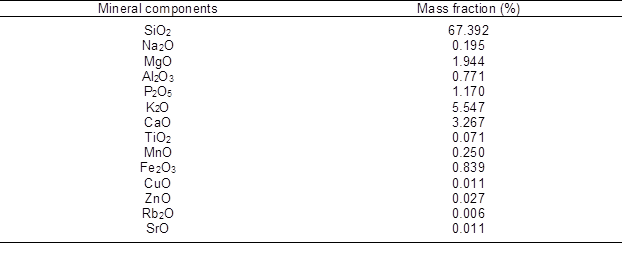Preparation procedure for silica

Preparation of sodium silicate was performed by alkaline-heating. A YXQ-LS-50A (Shanghai Boxun Co. Ltd., China) high-pressure reactor was used for mixing the CSA and sodium hydroxide solution. The mixed solution was washed with distilled water after steaming for a certain period (2 h, 4 h, 6 h, and 8 h) in the reactor. Sodium silicate was the filter liquor, which was separated from the mixed solution by a 2XZ(S)-2 (Shanghai Qianjing Pump Manufacturing Co. Ltd., China) sliding vane rotary vacuum pump.

Preparation of silica was performed by precipitation. A certain concentration of sodium silicate (5 °Bé, 10 °Bé, 15 °Bé, and 20 °Bé), a small amount of glycerol (0.67% of sodium silicate mass), and EDTA-Na(2.00% of sodium silicate mass) were added to a beaker and mixed by a RW20 digital agitator (Shanghai Chubai Laboratory Equipment Co. Ltd., China). This mixed liquid was aged for about 30 min at 70 to 90 °C. Sulfate acid was added to the mixed liquid at a given speed (0.27 mL/(min·100 mL)) with stirring until pH reached 7 to 10. Then, the beaker was left standing for 1 to 5 h, after which the silica was collected via centrifuging (16500 rpm) with a TG1650-WS high-speed centrifuge (Shanghai JL Optics Instrument Co., Ltd., China), scrubbed with distilled water, and dried in a DHG-9075A blast drying oven (Shanghai Yiheng Scientific Instrument Co. Ltd., China) at 105±1 °C.

Dissolution rate of silica

The dissolution rate of silica is expressed as the chemical reaction conversion rate of silica from CSA which reacts with the sodium hydroxide solution. Table 1 shows that the silica mass fraction of CSA is 67.329%. Hence, the dissolution rate of silica can be represented in Eq. 1: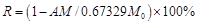(1)

where R represents the dissolution rate of silica (%); M0 is the weight of CSA before the chemical reaction (g); is the weight of residual solids after the chemical reaction (g); and A is the silica mass fraction of residual solids (%). A was determined by the X-ray fluorescence (XRF) method in the testing and analysis center of HIT.

Sodium silicate modulus

About 10 g of sodium silicate was dissolved in distilled water in a 200-mL volumetric flask; 10 mL of the solution was placed in a clean plastic cup with 50 mL of distilled water, and two drops of bromocresol green-methyl red mixed indicator were added to the solution. Hydrochloric acid (0.5 M) was dripped slowly into the mixture until the color changed from green to red. The time of the color change was denoted as point 1, at which point the volume of hydrochloric acid (V1) consumption was recorded. Next, 40 mL of sodium fluoride solution with a mass concentration of 48 g/L was added to the mixture to change the color once again to green. The 0.5 M hydrochloric acid was slowly added until the color of the solution in the cup changed from green to pink. This time was denoted as point 2, and the volume of hydrochloric acid (V2) consumption was noted. Thus, the sodium silicate modulus (N) was calculated using Eq. 2 (Huang 2005):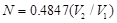(2)

The sodium silicate modulus (N) is an important factor in the production of precipitated silica from sodium silicate. In this work, Nis an aspect of comprehensive assessment for producing sodium silicate with CSA and sodium hydroxide solution.

Orthogonal experiment design

After fully considering the effects of various factors, the selection range of factor levels for the orthogonal experiment were identified and the best technological parameters for the preparation of the sodium silicate from CSA were obtained through experimentation. The orthogonal experiment design used an L16 (4)4 orthogonal table with four factors and four levels (Ren 1987; Li et al. 2007; Ilyas et al. 2012; Zhou et al. 2012), as listed in Table 2.

Table 2. Factors and Levels for L16 (4)4 Orthogonal Experiment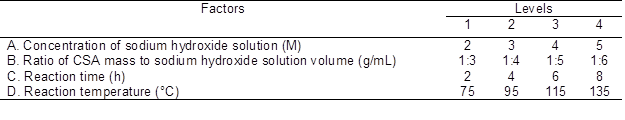Scoring standard and method of orthogonal experiment

The dissolution rate of silica and the sodium silicate modulus were the two indices used for a quality evaluation of the sodium silicate production from CSA. Hence, this orthogonal test is a multi-index test. There are two analysis methods for the multi-index test: comprehensive scoring and comprehensive balance methods. In the present study, the comprehensive scoring method was used because comprehensive scoring analysis is one index which changes from several indices (Zeng and Ye 2005; Liu et al. 2008).

In the comprehensive scoring method, a scoring standard should be set based on the actual test situation. According to the schemes of the orthogonal test, the scoring standard for the dissolution rate is the dissolution rate of silica multiplied by 100. Ding et al. (2009) previously found that 3.31 (N = 3.31) is the best modulus value for the production of precipitated silica from sodium silicate. Hence, the score was 100 when the modulus value of sodium silicate was 3.31, and 0 when the modulus value of sodium silicate was 1.31, the minimum modulus value of sodium silicate from the orthogonal test; the score should be reduced to 50 when the value difference between modulus value and 3.31 is 1. Therefore, the formula of comprehensive scoring can be expressed as follows,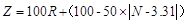(3)

where Z is the value of comprehensive scoring; represents the dissolution rate of silica (%); and is the sodium silicate modulus.

Statistical method of range analysis

The range analysis of orthogonal experiment was analyzed using the statistical method which was studied by Zhu (2011), as listed in Table 3.

Table 3. Statistical method of range analysis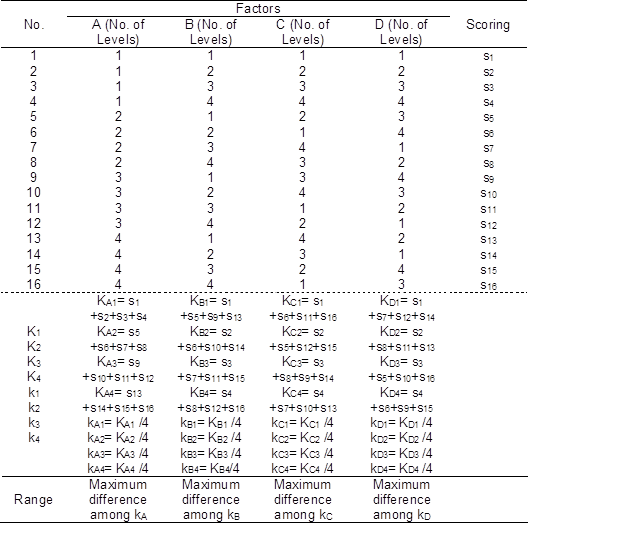Characteristics of silica

The purities of the as-obtained silica products were tested by improved silicon-molybdenum blue spectrophotometry (Ding et al. 2009). The HG/T3061 (2009) standard was used to estimate whether the as-obtained silica products reached market requirements. Fourier transform infrared spectroscopy (FT-IR) of the as-obtained silica products was analyzed with a VERTEX 70 Fourier transform infrared spectrometer (Germany) by KBr squash method. The surface microstructure of the silica products were characterized by an FEI Quanta 200 FEG scanning electron microscope (SEM) (USA) operating at an accelerating voltage of 30 kV. The samples were attached to an aluminum stub with tape and sputter-coated with gold. The surface ultrastructure of the samples was characterized with an Hitachi H-7650 transmission electron microscope (TEM) (Japan) operating at an accelerating voltage of 120 kV. The samples were sonicated in ethanol to disperse and dried on a copper grid. The BET surface area of the silica products was tested by a JW-BK132F static nitrogen adsorption instrument (Beijing JWGB Sci.&Tech. Co. Ltd., China). Samples were pre-treated for 5 h at 200 °C and a vacuum degree of -10 kPa. Then the surface area can be calculated by BET method and most probable pore size can be calculated by BJH model.

RESULTS AND DISCUSSION

Effect of Single Factors on the Preparation of Sodium Silicate

The effects of the concentration of sodium hydroxide solution, the ratio of CSA mass to sodium hydroxide solution volume, reaction time, and reaction temperature on the preparation of sodium silicate are shown in Fig. 1.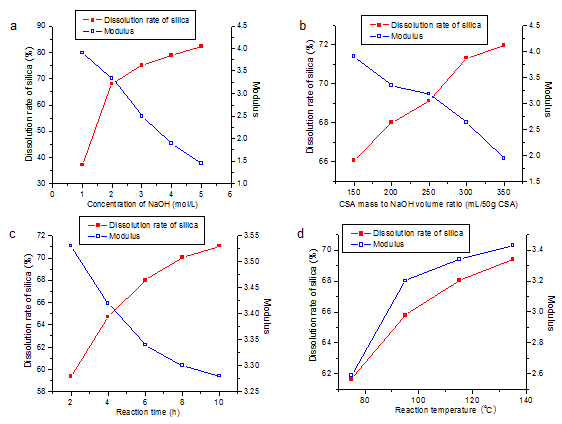Fig.1. Effects of various factors on the dissolution rate of silica and sodium silicate modulus: (a) effect of concentration of sodium hydroxide solution; (b) effect of the ratio of CSA mass to sodium hydroxide solution volume; (c) effect of reaction time; and (d) effect of reaction temperature

Effect of concentration of sodium hydroxide solution

The effect of the concentration of sodium hydroxide solution on the dissolution rate of silica and sodium silicate modulus was examined under the following conditions: the ratio of CSA mass to sodium hydroxide solution volume was 50 g:250 mL, the reaction time was 6 h, and the reaction temperature was 115 °C. The results are shown in Fig. 1a. The dissolution rate of silica increased with increasing concentration of sodium hydroxide solution, but the change in the dissolution rate of silica was not obvious when the concentration of sodium hydroxide solution was over 3 M (Fig. 1a). At the same time, the sodium silicate modulus was reduced with increasing concentration of the sodium hydroxide solution. Because of the increase in the concentration of the sodium hydroxide solution, the alkali content that came into contact with the silica from CSA increased, so the dissolution rate of silica increased; but the increase in the alkali content resulted in a decrease in the sodium silicate modulus. Therefore, a high concentration of sodium hydroxide solution may be chosen for increasing the dissolution rate of silica, and a low concentration of sodium hydroxide solution can be used to increase the sodium silicate modulus. The concentration of sodium hydroxide solution should be adjusted for different sodium silicate moduli in actual production.

Effect of the ratio of CSA mass to sodium hydroxide solution volume

The effect of the ratio of CSA mass to sodium hydroxide solution volume for the dissolution rate of silica and sodium silicate modulus was studied when the concentration of sodium hydroxide solution was 2 M, the reaction time was 6 h, and the reaction temperature was 115 °C. The results are shown in Fig. 1b. The dissolution rate of silica increased and sodium silicate modulus was reduced with increasing sodium hydroxide volume (Fig. 1b). In the reaction system, when the relative content of CSA was large and the relative content of sodium hydroxide was small, the reaction with silica could not adequatelyinfiltrate the pores of CSA, i.e., the dissolution rate of the silica was small. The sodium silicate modulus, however, was not small because the actual content of sodium hydroxide was small. With increasing amounts of sodium hydroxide, the dissolution rate of silica increased, although the increase in the dissolution rate of silica was not large (66.07% to 71.98%), and the sodium silicate modulus was noticeably reduced (3.90 to 1.94).

Effect of reaction time

The effect of reaction time on the dissolution rate of silica and sodium silicate modulus was investigated when the concentration of sodium hydroxide solution was 2 M, the ratio of CSA mass to sodium hydroxide solution volume was 50 g:250 mL, and the reaction temperature was 115 °C. The results are shown in Fig. 1c. The dissolution rate of silica increased and sodium silicate modulus was reduced with increasing reaction time (Fig. 1c). It can be seen that the increase in the dissolution rate of silica and the decrease in the sodium silicate modulus changed slightly after 6 h. The energy consumption was larger with more reaction time from an economic perspective. In fact, 6 h is apparently a suitable reaction time.

Effect of reaction temperature

The effect of reaction temperature on the dissolution rate of silica and sodium silicate modulus was examined when the concentration of sodium hydroxide solution was 2 M, the ratio of CSA mass to sodium hydroxide solution volume was 50 g:250 mL, and the reaction time was 6 h. The results are shown in Fig. 1d. The dissolution rate of silica and the sodium silicate modulus increased with increasing reaction temperature (Fig. 1d). The dissolution rate of the silica and sodium silicate modulus increased slightly as the temperature increased above 115 °C. Energy consumption was larger with higher reaction temperature from an economic perspective. This should thereby be considered comprehensively in actual production.

Optimization of Technological Parameters for the Preparation of Sodium Silicate

The schemes and results of the orthogonal experiment are shown in Table 4.

Table 4. Schemes and Results of Orthogonal Experiment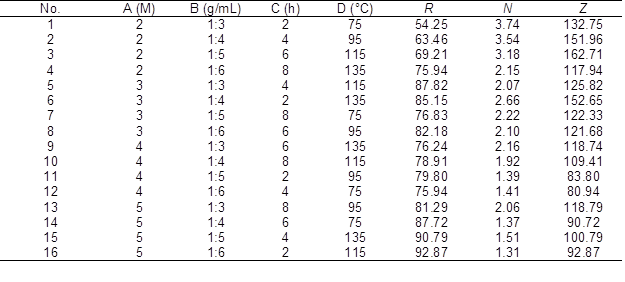Range analysis of orthogonal experiment

Range analysis was performed according to the results of comprehensive scoring (Z) in Table 4. The comprehensive index was analyzed using the statistical method. The range analysis results for the orthogonal experiment are presented in Table 5.

Table 5 shows that the range of the concentration of sodium hydroxide solution, factor A, was maximal. Hence, the comprehensive influence of factor A on the dissolution rate of silica and the sodium silicate modulus was maximal.

Table 5. Range Analysis Results of Orthogonal Experiment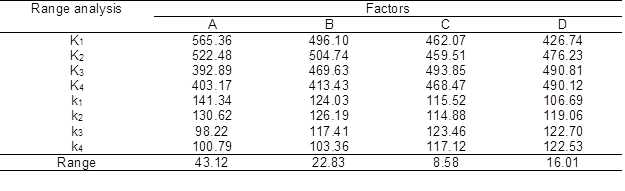The comprehensive influence levels of the other factors from high to low were factor B, the ratio of CSA mass to sodium hydroxide solution volume, factor D, reaction temperature, and factor C, reaction time. For each factor, the comprehensive indices of different levels are shown in Fig. 2 according to the results in Table 5. Thus, one can confirm the best level for each factor directly for the production of sodium silicate from CSA.

Figure 2 shows that the best scheme was A1B2C3D3. Therefore, the optimum reaction conditions for the production of sodium silicate from CSA are: A = 2 M, B = 1:4 g/mL, C = 6 h, and D = 115 °C.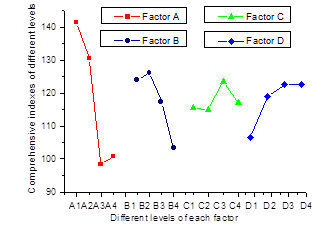Fig. 2. Comprehensive indices at different levels for each factor

Variance analysis of orthogonal experiment

The sum of squares of deviations (Q) for each factor is calculated first, followed by the degree of freedom (f), mean square (MS), and value of F testing method (F-value) of each factor determined. Next, the F-value should be checked when the test level is given. The computational method can be expressed as follows (He et al. 2002; Jiet al. 2010).

a. Sum of squares of deviations (Q):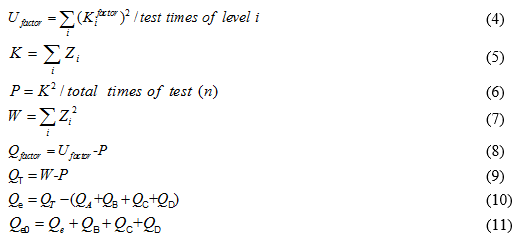In these formulas, Kfactor and Zi come from the data in Table 5. QfactorQTQe, and Qe0 represent the sum of the squares of deviations for each factor, total, error, and correcting error, respectively.

b. Degree of freedom (f):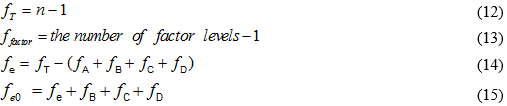where fTffactor, feand fe0 represent the degree of freedom for total, each factor, error, and correcting error, respectively.

c. Mean square (MS):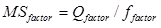(16)

where MSfactor represents the mean square of each factor.

d. F-value: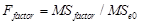(17)

e. Method for distinguishing the significant influence factor:

If Ffactor>Fα (ffactor, fe0), this factor is a significant influence factor for the test result. The value of α is 0.05.

The results of the variance analysis are shown in Table 6 according to the above method.

Table 6. Results of Variance Analysis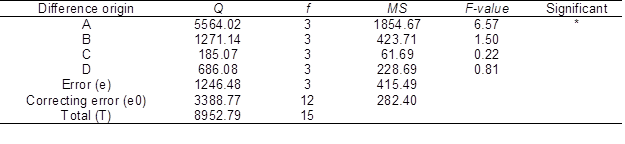* α = 0.05

It can be seen that FF0.05 (3, 12) = 3.49, FBFCF< 3.49. Hence, factor A was a significant influence factor, whereas factors B, C, and D were not. Table 5 also shows that the sequence of factors affecting the test was A > B > D > C, which is consistent with the results of the range analysis.

Verification of the best scheme

The best scheme, A1B2C3D3, was not performed according to the above analysis. Hence, it should be tested based on the above analysis. The best results show that the dissolution rate of silica was 68.01%, the sodium silicate modulus was 3.34, and the comprehensive scoring was 166.51 when this scheme was used.

Effect of Single Factor for the Preparation of Silica

The effects of the concentration of sodium silicate, the concentration of sulfate, the reaction temperature, and aging time on the purity of silica were studied (Fig. 3). The above sodium silicate with a modulus of 3.34 was used as the raw material for the preparation of silica. In the preparation process of silica, the addition quantity of EDTA-Na2 was set as 2.0%, the additional quantity of glycerol was 0.67%, the adding speed of sulfate was 0.27 mL/(min·100mL), and pH was 8 at the end of the experiment. The purities of silica under different stirring speeds were determined by improved silicon-molybdenum blue spectrophotometry.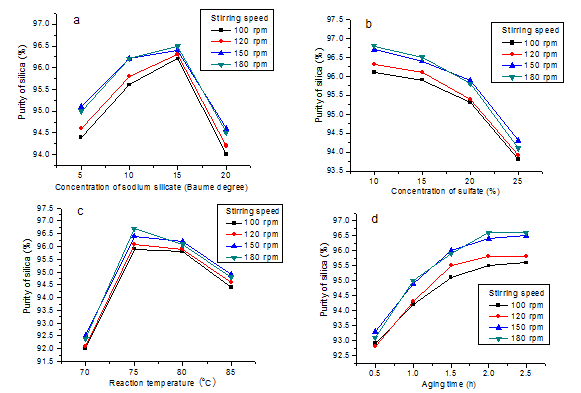Fig. 3. Effects of various factors on the purity of silica: (a) effect of concentration of sodium silicate; (b) effect of concentration of sulfate; (c) effect of reaction temperature; and (d) effect of aging time

Effect of concentration of sodium silicate

The effect of the concentration of sodium silicate on the purity of silica was studied when the concentration of sulfate was 15%, the reaction temperature was 75 °C, and the aging time was 2 h. The results are shown in Fig. 3a. The purity of silica first increased with increasing sodium silica concentration and then decreased (Fig. 3a). Because the relative content of SiO2 in sodium silicate increased with increasing sodium concentration and, according to the precipitation theory which was studied by Yu (1997), the purity of the silica was first increased and then reduced with increasing content of SiO2 in the sodium silicate. It is therefore suggested that 15 °Bé is the most suitable concentration value of sodium silicate for obtaining silica with high purity.

Effect of concentration of sulfate

The effect of the concentration of sulfate on the purity of silica was investigated when the concentration of sodium silicate was 15 °Bé, the reaction temperature was 75 °C, and the aging time was 2 h. The results are shown in Fig. 3b. The purity of silica was reduced with increasing concentration of sulfate (Fig. 3b). The pH changed quickly when the concentration of sulfate was high, so the amount of particle products is relatively large per unit time and more impurities are encapsulated because there are many opportunities for aggregation. The pH changed slowly when the concentration of sulfate was low, so the amount of particle products was small per unit time and the aggregation opportunity of particles was small; because the concentration of sulfate was low, the volume of sulfate solution added to the beaker to reach a neutral pH value was large. Therefore, the volume of the final solution was large, the probability of particle aggregation was small, and the purity of the silica was high. Increased reaction time, however, results in greater energy consumption when the concentration of sulfate is low. Comprehensive consideration suggests that the suitable concentration value of sulfate is 15%.

Effect of reaction temperature

The effect of reaction temperature on the purity of silica was also researched when the concentration of sodium silicate was 15 °Bé, the concentration of sulfate was 15%, and the aging time was 2 h. The results are shown in Fig. 3c. The purity of silica is increased first and then reduced with increasing reaction temperature (Fig. 3c). The liquid was paste cluster, and the precipitate could not be separated from the liquid when the reaction temperature was below 60 °C. Sodium silicate viscosity was high when the reaction temperature was low, so the probability of particle aggregation was small, and finally, the liquid became a silica sol system. With increasing reaction temperature, sodium silicate viscosity was reduced, the probability of particle aggregation increased, and the silica sol dissolved gradually. Aggregation speed, however, was very quick and more impurities were encapsulated following the continual increase in reaction temperature, so the resulting silica had lower purity. Comprehensive consideration suggests that 75 °C is a suitable reaction temperature.

Effect of aging time

The effect of aging time on the purity of silica was examined when the concentration of sodium silicate was 15 °Bé, the concentration of sulfate was 15%, and the reaction temperature was 75 °C. The results are shown in Fig. 3d. The purity of silica increased with increasing aging time (Fig. 3d). The purity increase, however, became progressively smaller. At the same time, the increase in aging time reduced the production capability. Therefore, 2 h is suggested as a reasonable aging time.

Characteristics of Silica Products

Comparison of HG/T3061 (2009) standards and characteristics of silica products

After testing some characteristics of the silica products, comparison of HG/T3061 (2009) standards and testing results of the silica products were used to estimate whether the as-obtained silica products reached market requirements. The results are shown in Table 7.

Table 7. HG/T3061 (2009) Standards and Characteristics of Silica Products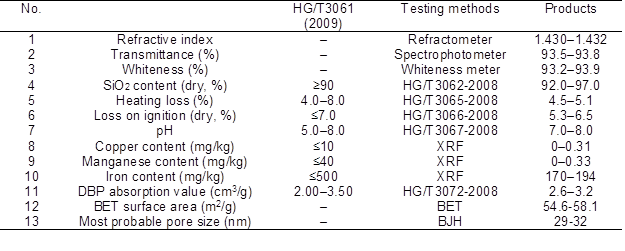Table 7 shows that various indices of silica products were superior to the standard requirements, and the as-obtained silica products can be applied to the market field.

FT-IR of the as-obtained silica

Fourier transform infrared spectroscopy (FT-IR) of the as-obtained silica was analyzed with a VERTEX 70 Fourier transform infrared spectrometer by KBr squash method. The results are shown in Fig. 4.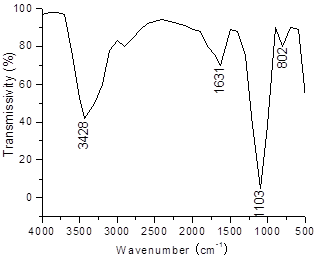Fig. 4. FT-IR analysis of the as-obtained silica

Figure 4 shows that the as-obtained silica had characteristic IR absorption peaks of typical hydrated silicon dioxide. The peaks at 3428 cm-1, 1631 cm-1, 1103 cm-1 and 802 cm-1 represent stretching vibration peak of Si-OH, bending vibration peak of O-H (from crystal water in the as-obtained silica), asymmetric stretching vibration peak of Si-O-Si and symmetrical stretching vibration peak of Si-O-Si, respectively.

Microstructure and ultrastructure of the as-obtained silica

The microstructure of the as-obtained silica product was characterized by SEM and the ultrastructure by TEM. The results are shown in Fig. 5.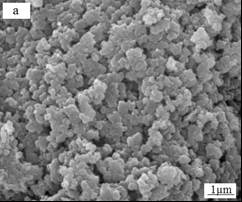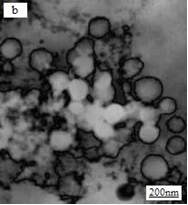Fig. 5. (a) The microstructure of the as-obtained product sample by SEM and (b) the ultrastructure of the as-obtained product sample by TEM

Figure 5a shows that the as-obtained silica product was a cluster that formed from the polymerization of a great number of SiO2 particles. These SiO2 particles are nano-scale particles, and the size of each particle is less than 50 nm (Fig. 5b). Nano-scale silica is very useful and expensive, so the above preparation procedure of silica from CSA has a large market potential.

CONCLUSIONS

1. The concentration of sodium hydroxide solution was confirmed to have a significant influence on the production of sodium silicate from CSA, and the optimum technological parameters for the preparation of sodium silicate are: concentration of sodium hydroxide solution = 2 M, ratio of CSA mass to sodium hydroxide solution volume = 1:4 g/mL, reaction time = 6 h, and reaction temperature = 115 °C.
2. The following parameters are suitable for the preparation of silica: concentration of sodium silicate = 15 °Bé, concentration of sulfate = 15%, reaction temperature = 75 °C, and aging time = 2 h.
3. The as-obtained silica product has characteristic ir absorption peaks of typical hydrated silicon dioxide, and it is a cluster formed from nano-scale SiO2 particles. Various indices of the silica product are superior to the standard requirements, so the as-obtained silica product can be applied commercially.

ACKNOWLEDGEMENTS

This work was supported by the National Development and Reform Commission of China (Resource Conservation and Environmental Protection Project: Comprehensive Utilization of Hybrid Fuel Ash).

REFERENCES CITED

An, D. M., Guo, Y. P., Zhu, Y. C., and Wang, Z. C. (2010). “A green route to preparation of silica powders with rice husk ash and waste gas,” Chem. Eng. J. 162(2), 509-514.

Binici, H. F., and Aksogan, O. (2011). “The use of ground blast furnace slag, chrome slag and corn stem ash mixture as a coating against corrosion,” Construct. Build. Mater. 25(11), 4197-4201.

Ding, K. Y., Zhang, Y. J., and Liu, C. M. (2009). “Influence factors for production of high purity silica and sodium silicate from rice husk ash,” J. Ch. Cereal. Oils Assoc. 24(5), 1-6.

Gao, P. J., Qu, Y. B., Zhao, X., Zhu, M. T., and Duan, Y. C. (1997). “Screening microbial strain for improving the nutritional value of wheat and corn straws as animal feed,” Enzyme Microb. Technol.20(8), 581-584.

He, S. H., Wen, Z. Q., and Lou, T. (2002). Experiments Design and Data ProcessingNUDT Press, Changsha, pp. 62-101.

HG/T3061. (2009). Rubber compounding ingredients. Silica, precipitated, hydrated, CCBI. ISO 5794-1-2005, MOD.

Huang, C. H. (2005). “Improvement for rapid determination of the modulus of sodium silicate,” PTCA (Part B: Chem. Anal.) 41(4), 281.

Huang, H. Y., Chang, Z. Z., and Ma, Y. (2006). “Production of elements fertilizer from straw ash,” CN 1793074A, China.

Ilyas, S., Chi, R. A., Lee, J. C., and Bhatti, H. N. (2012). “One step bioleaching of sulphide ore with low concentration of arsenic by Aspergillus niger and Taguchi orthogonal array optimization,” Chin. J. Chem. Eng. 20(5), 923-929.

Ji, Y. Z., Hu, W., and Zhao, Y. L. (2010). “Confirmation of the optimum technology for extraction of rhubarb polysaccharide by the variance analysis method,” J. CUT. 31(2), 132-136.

Ju, S. B. (2010). “Technology analysis of extracting potash from power plant straw ash,” Henan Chem. Ind. 27(3), 18-20.

Kohls, D. J., and Beaucage, G. (2002). “Rational design of reinforced rubber,” Sol. Sta. Mater. Sci. 6(3), 183-194.

Li, B. Y. (2010). “Status quo and development trend of domestic and overseas precipitated silica,” CHN Rubb. 26(24), 9-15.

Li, J., Huang, J. X., Zhao, J. D., and Qu, Y. (2011). “Development status and market prospect of white carbon black,” Sci. Technol. Chem. Ind. 19(4), 67-71.

Li, Y., Zhang, Y. P., and Zhang, R. Q. (2007). “Production of phosphorus potassium compound fertilizer from straw ash in straw power plant,” CN 1911867A, China.

Li, Y. F., and Wu, X. M. (2012). “Development status and prospect of silica at home and abroad,” Fine Chem. Ind. Raw Mater. Inter. 11(7), 20-23.

Li, Y. F., Yan, X. H., Jiang, G. F., Liu, Q., Song, J. X., and Guo, C. C. (2007). “Toluene oxyfunctionalization with air over metalloporphyrins and reaction condition optimization,” Chin. J. Chem. Eng. 15(3), 453-457.

Li, Z. Q., Zhao, W., Meng, B. H., Liu, C. L., Zhu, Q. Y., and Zhao, G. B. (2008). “Kinetic study of corn straw pyrolysis: Comparison of two different three-pseudocomponent models,” Bioresour. Technol. 99(16), 7616-7622.

Liu, J., Hou, S. E., Qi, H. Y., and Xiao, H. Y. (2008). “Orthogonal test for production of sodium silicate from rice husk ash,” Cere. Feed Ind. 30(12), 6-10.

Liu, Y., Guo, Y.P., Zhu, Y.C., An, D.M., Gao, W., Wang, Z., Ma, Y.J., Wang, Z.C. (2011). “A sustainable route for the preparation of activated carbon and silica from rice husk ash,” J. Hazard. Mater.186(2-3), 1314-1319.

Liu, Y., Guo, Y.P., Gao, W., Wang, Z., Ma, Y.J., Wang, Z.C. (2012). “Simultaneous preparation of silica and activated carbon from rice husk ash,” J. Clean. Product. 32, 204-209.

Ren, L. Q. (1987). Test Optimization Technique, China Machine Press, Beijing, pp. 5-32.

Xu, X. S., Feng, P. Z., and Zhang, L. Q. (2008). “Separation method of potassium salt from straw ash,” CN 101172889A, China.

Yan, D. Z., Tang, C. B., and Xie, Z. H. (2010). “Study on production technology of fumed silica,” Energy Saving Non-Ferro. Metal. 12(6), 33-35.

Yu, H. Y. (1997). Grain Processing Technology, China Financial and Economic Publishing House, Beijing, pp. 7.

Yue, Q., and Jiang, S. F. (2009). “Experiments of the slag cement admixture with corn straw ash,” Cement. 19(11), 4-5.

Zeng, L. K., and Ye, W. P. (2005). The Applications of Computer for Material Science and EngineeringWuhan University of Technology Press, Hubei, pp. 1-24.

Zhang, T. T., Huang, J., Deng, S. B., and Yu, G. (2011). “Influence of pesticides contamination on the emission of PCDD/PCDF to the land from open burning of corn straws,” Environ. Pollut. 159(6), 1744-1748.

Zhang, Y. Z., Fu, X. G., and Chen, H. Z. (2012). “Pretreatment based on two-step steam explosion combined with an intermediate separation of fiber cells-Optimization of fermentation of corn strawhydrolysates,” Bioresour. Technol. 121, 100-104.

Zhao, W., Li, Z. Q., Wang, D. W., Zhu, Q. Y., Sun, R., Meng, B. H., and Zhao, G. B. (2008). “Combustion characteristics of different parts of corn straw and NO formation in a fixed bed,” Bioresour. Technol.99(8), 2956-2963.

Zhong, W. Z., Zhang, Z. Z., Luo, Y. J., Sun, S. S., Qiao, W., and Xiao, M. (2011). “Effect of biological pretreatments in enhancing corn straw biogas production,” Bioresour. Technol. 102, 11177-11182.

Zhou, Y. Y., Liu, H. B., Zou, Y. P., and Xie, H. (2012). “Optimization of conditions for microwave digesting treatment of facial cleaning milk by orthogonal experimental design,” Chin. Sur. Det. Cos. 42(4), 280-281.

Zhu, Y. Q. (2011). “Range analysis of orthogonal experiment based on easy language,” Bev. Ind. 14(2), 27-30.

Article submitted: May 14, 2014; Peer review completed: August 9, 2014; Revised version received and accepted: September 16, 2014; Published: September 23, 2014.